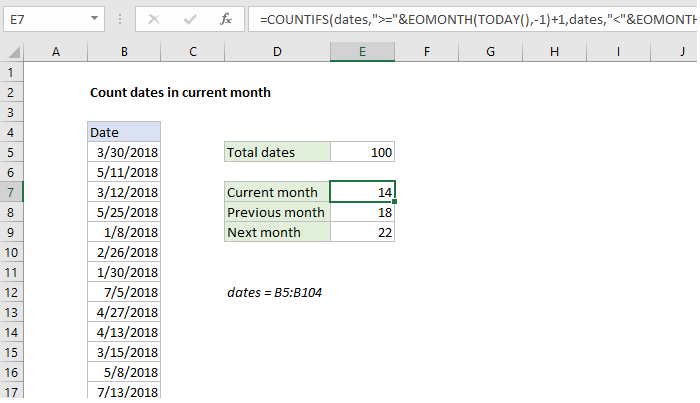## Excel Office

Excel How Tos, Tutorials, Tips & Tricks, Shortcuts

# Count dates in current month in Excel

This tutorial shows how to Count dates in current month in Excel using example below.

To count dates in the current month, you can use a formula based on the COUNTIFS or SUMPRODUCT function as explained below.

## Formula

```=COUNTIFS(range,">="&EOMONTH(TODAY(),-1)+1,
range,"<"&EOMONTH(TODAY(),0)+1)```## Explanation

In the example shown above, the formula in E7 is:

```=COUNTIFS(dates,">="&EOMONTH(TODAY(),
-1)+1,dates,"<"&EOMONTH(TODAY(),0)+1)```

Where “dates” is the named range B5:B104.

### How this formula works

At the core, this formula uses the COUNTIFS function to count dates in a range when the are greater than or equal to the first day of the current month, and less than the first day of the next month. The EOMONTH function is used to create both dates based on the current date, which is supplied by the TODAY function.

Worked Example:   How to calculate workdays per month in Excel

To get the first day of the month, we use:

`EOMONTH(TODAY(),-1)+1`

Here, the EOMONTH function returns the last day of the previous month, to which 1 is added to get the first day of the current month.

Worked Example:   Count occurrences in entire Excel Workbook

To get the last day of the current month, EOMONTH is used like this:

`EOMONTH(TODAY(),0)+1`

EOMONTH returns the last day of the current month, to which 1 is added to get the first day of the next month.

To count dates in the previous month:

```=COUNTIFS(dates,">="&EOMONTH(TODAY(),-2)
+1,dates,"<"&EOMONTH(TODAY(),-1)+1)```

To count dates in the next month:

```=COUNTIFS(dates,">="&EOMONTH(TODAY(),0)
+1,dates,"<"&EOMONTH(TODAY(),1)+1)```

### SUMPRODUCT alternative

You can also count dates in the previous, current, and next month using SUMPRODUCT like this:

```=SUMPRODUCT(N(TEXT(EOMONTH(TODAY(),-1),
"mmyy")=TEXT(range,"mmyy")))
=SUMPRODUCT(N(TEXT(TODAY(),"mmyy")=
TEXT(range,"mmyy")))
=SUMPRODUCT(N(TEXT(EOMONTH(TODAY(),1),
"mmyy")=TEXT(range,"mmyy")))```

Here, dates are fed into the TEXT function to get a month and year string, which is used for comparison in an array operation inside SUMPRODUCT. The result is an array of TRUE FALSE values, where TRUE represents dates in the month of interest.

Worked Example:   COUNTIFS function: Description, Usage, Syntax, Examples and Explanation

The N function is used to change these values to ones and zeros, and SUMPRODUCT simply sums and returns the array.